Examples

Chapter 7 Class 11 Permutations and Combinations (Term 2)
Serial order wise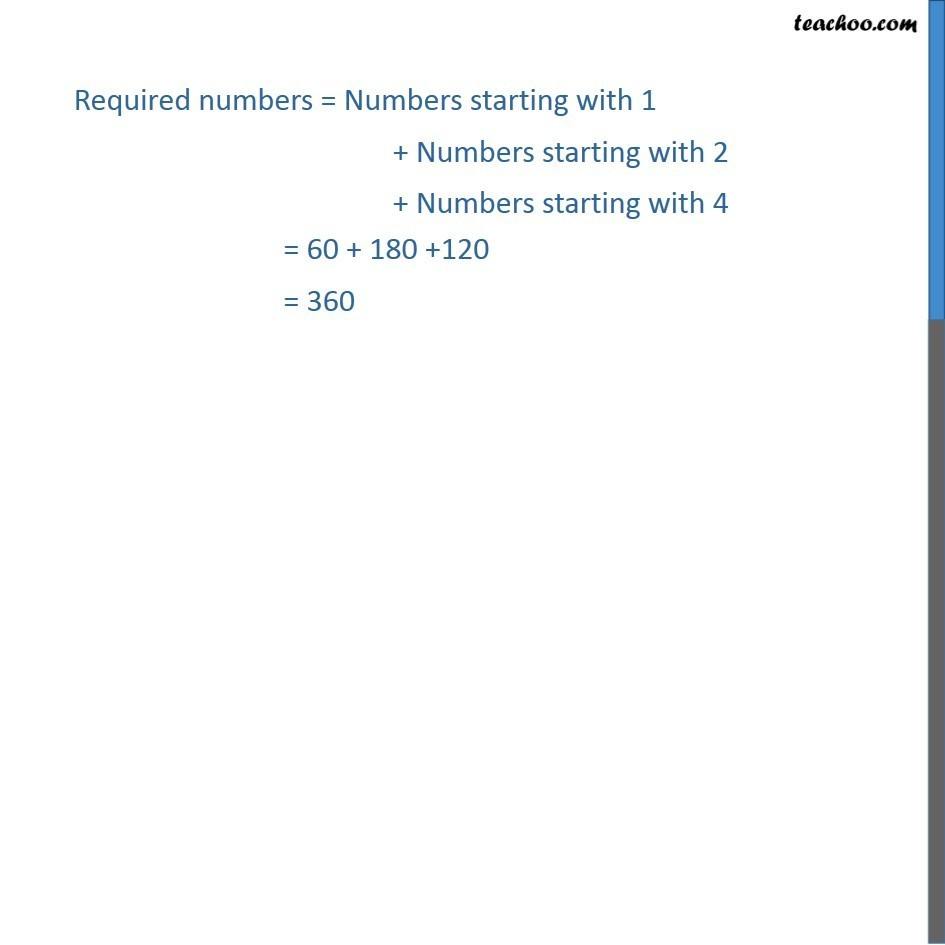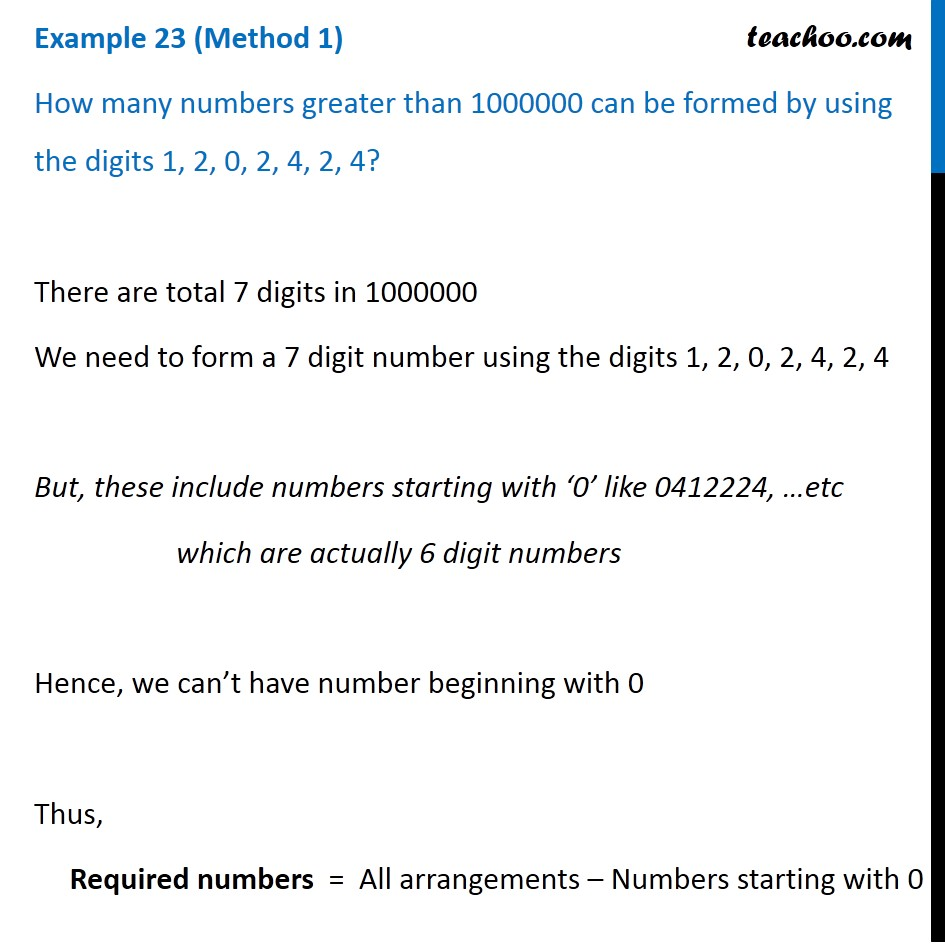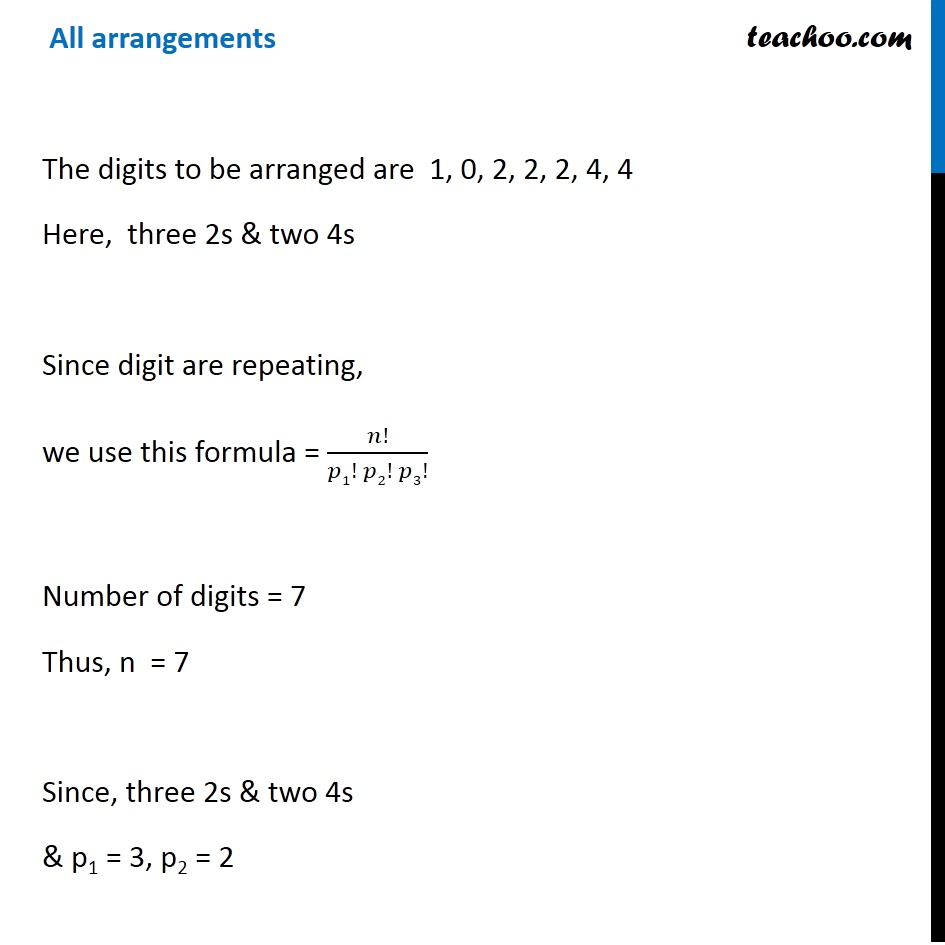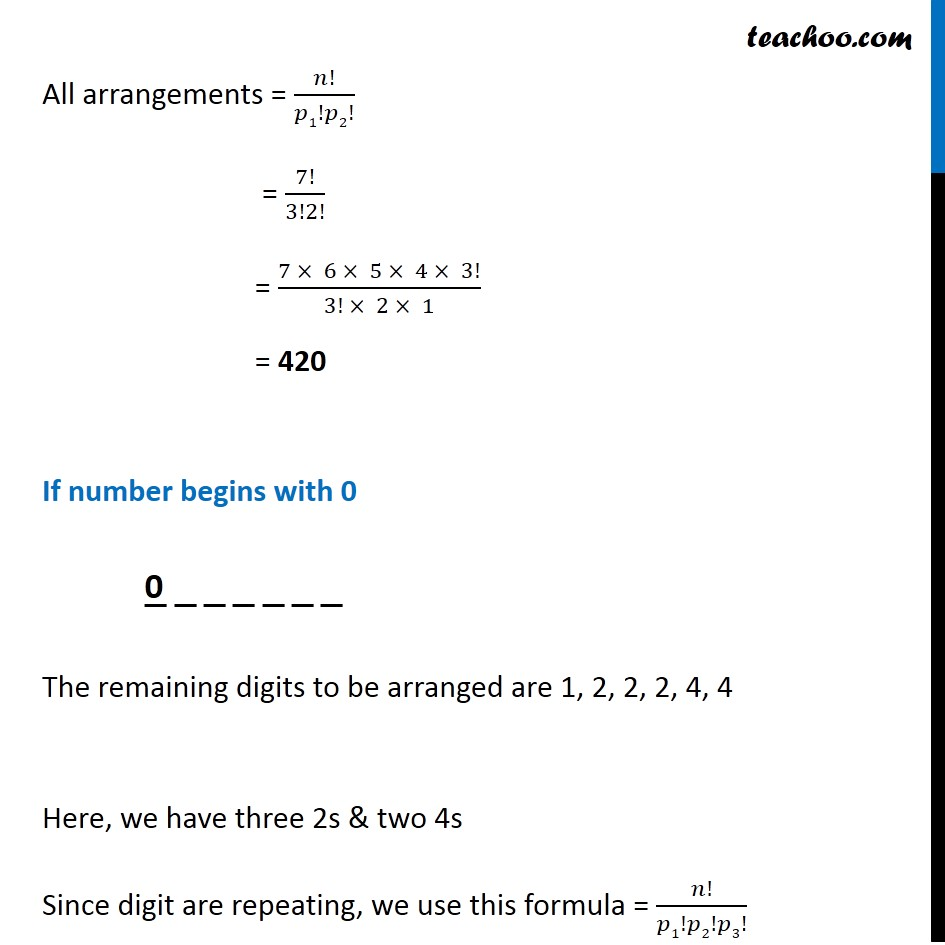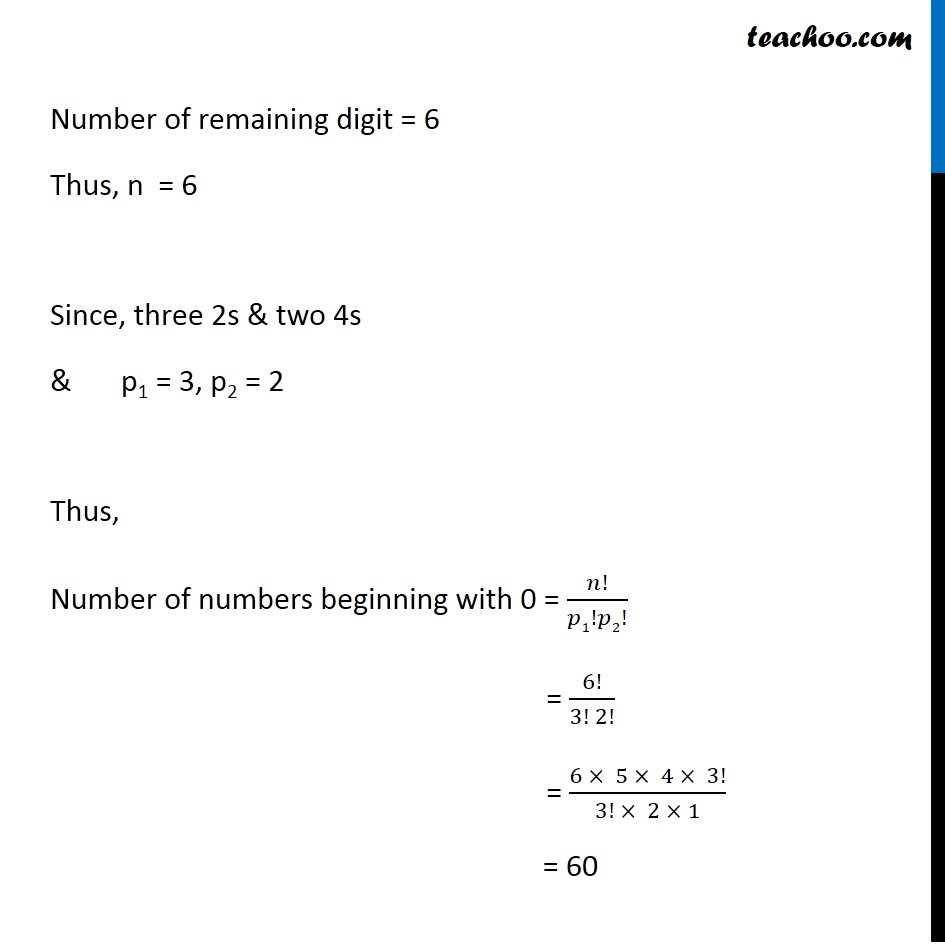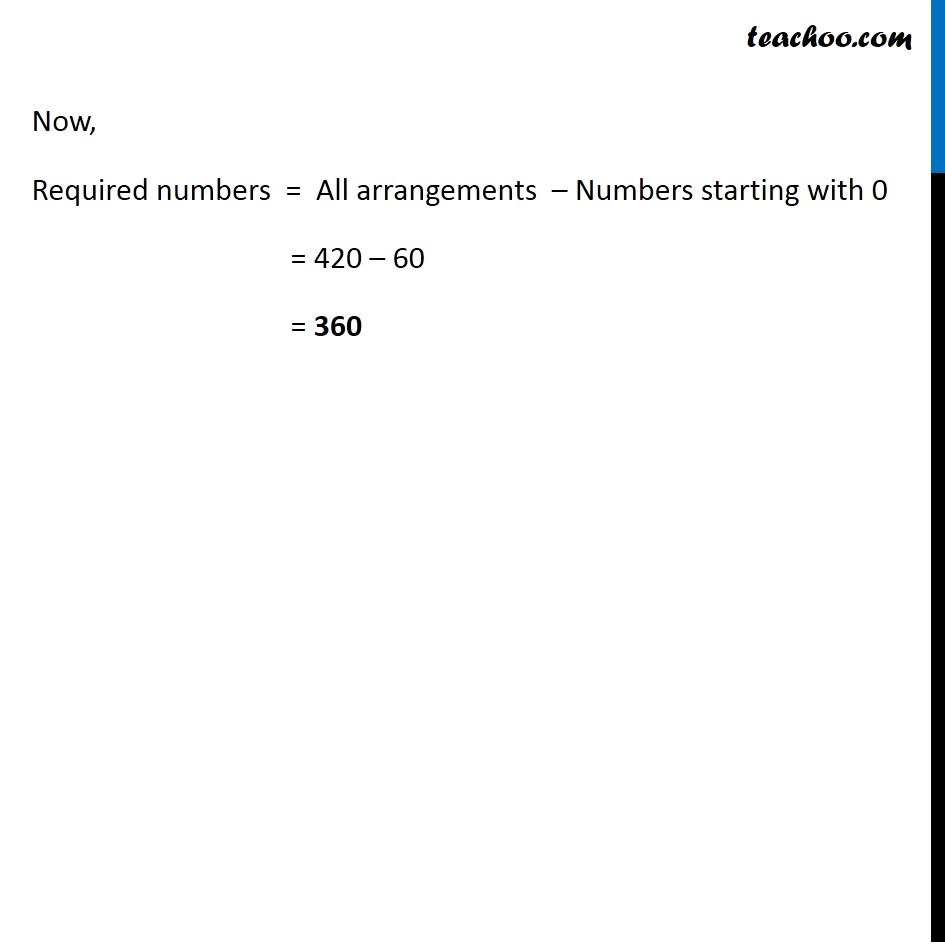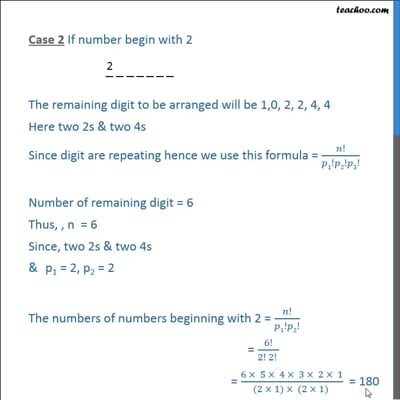This video is only available for Teachoo black users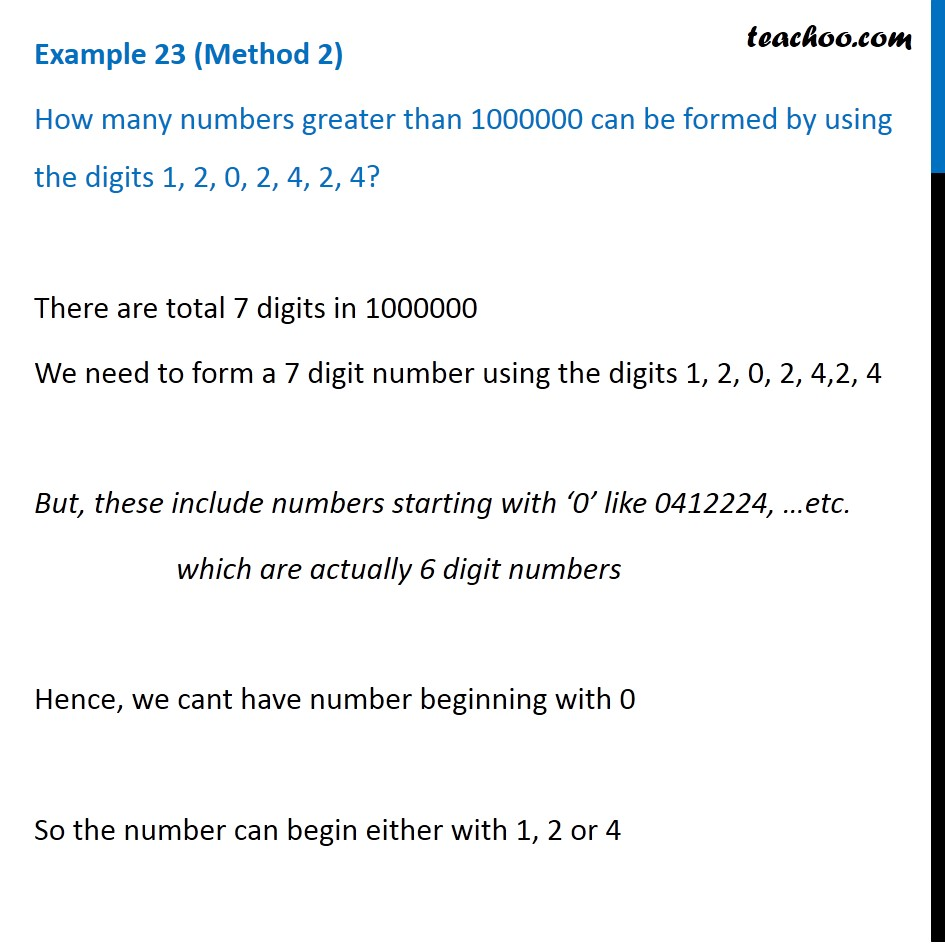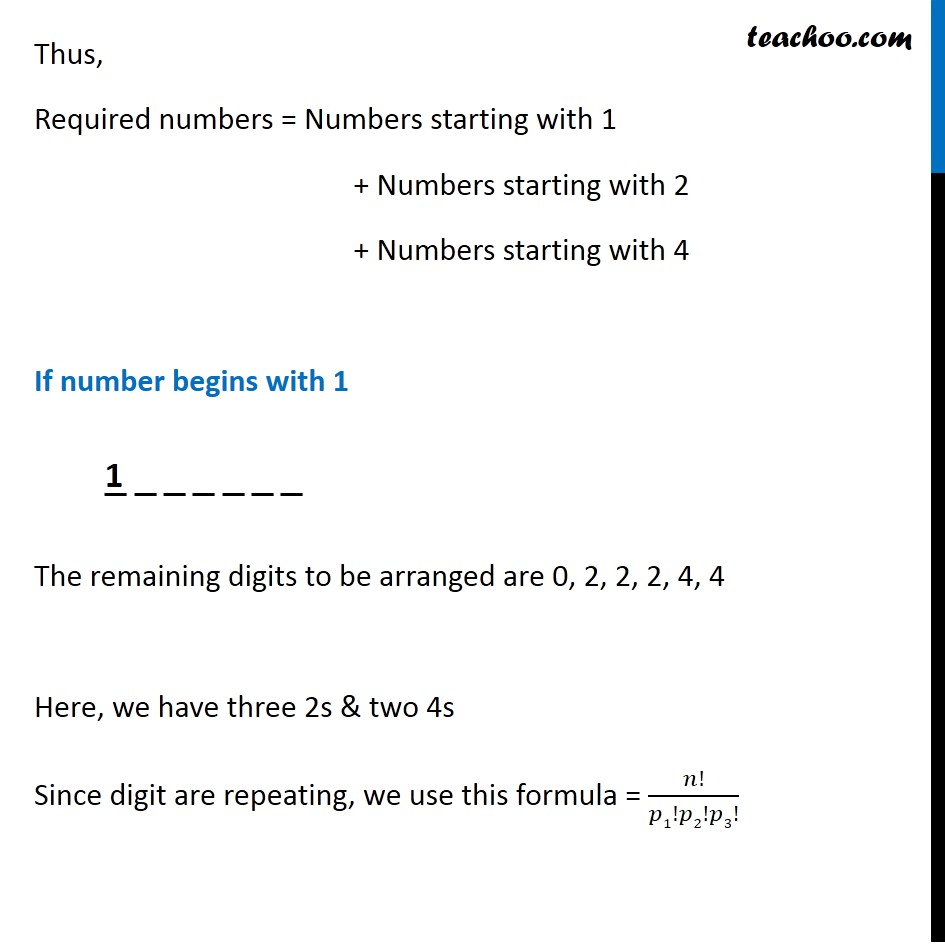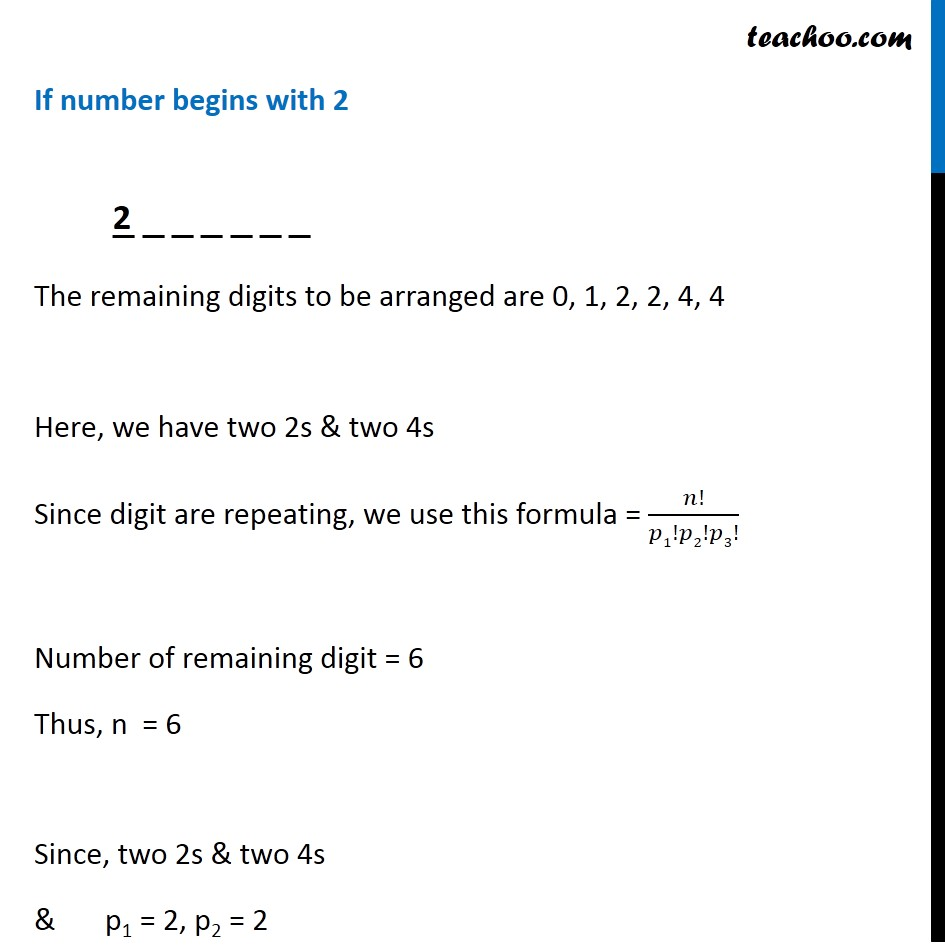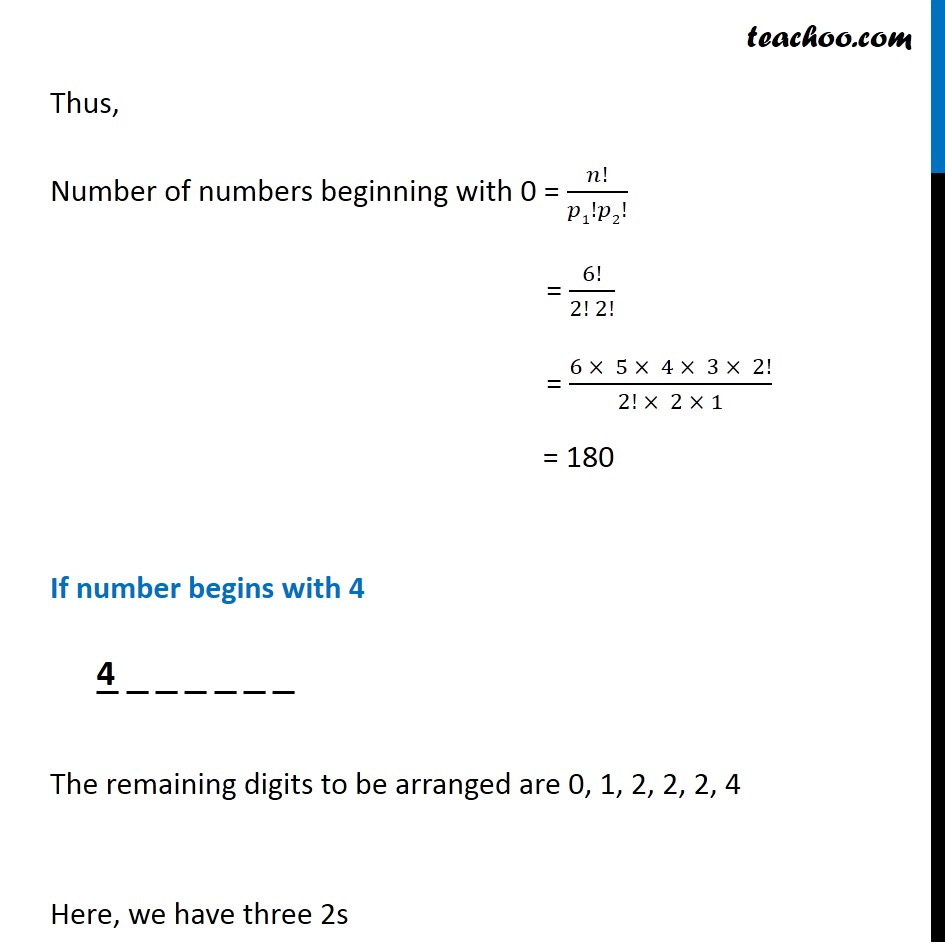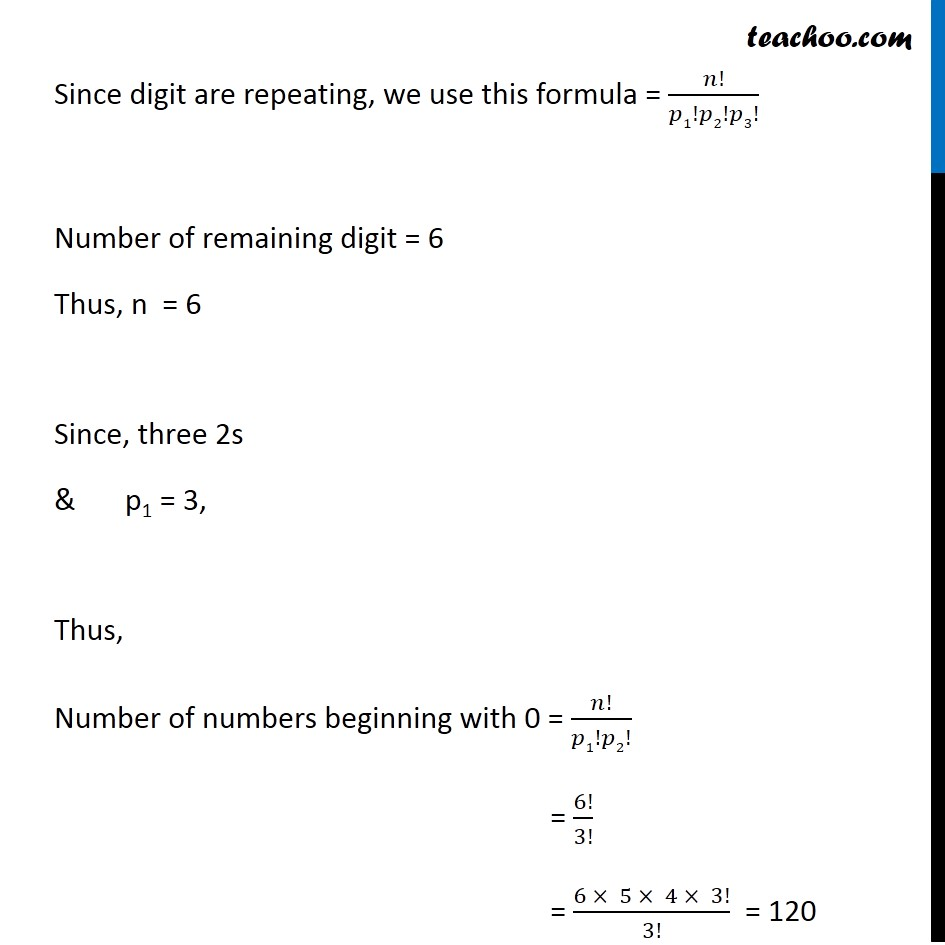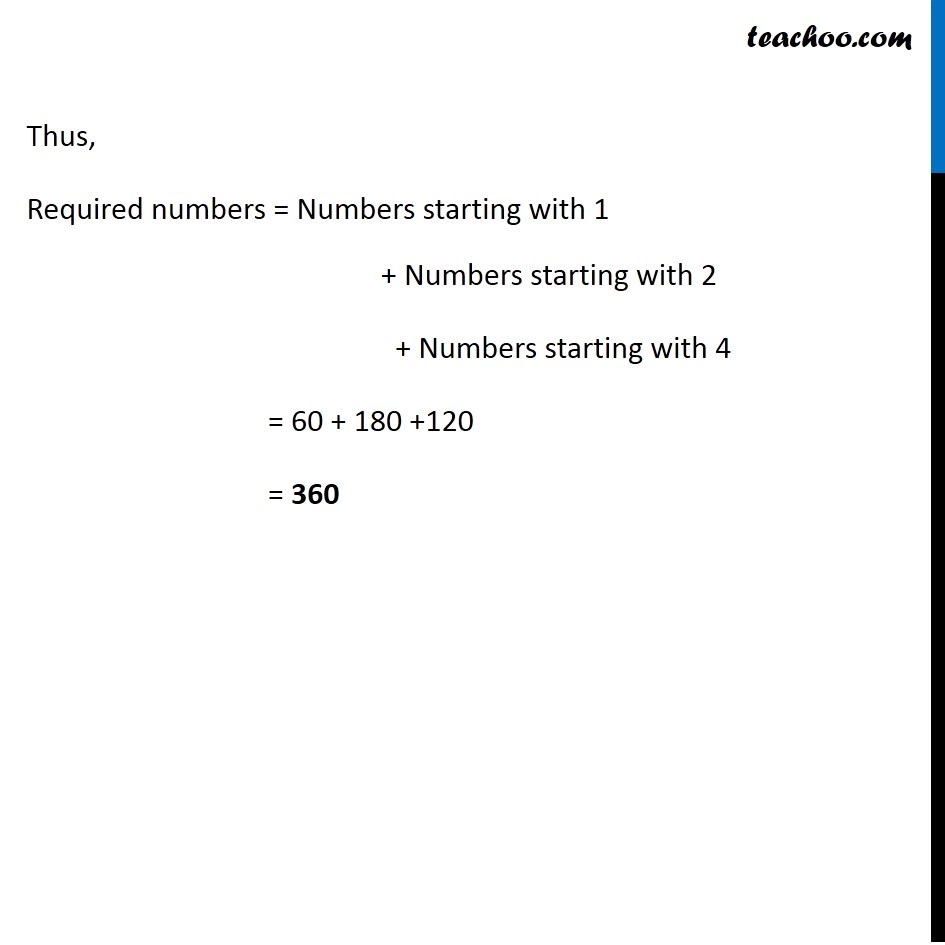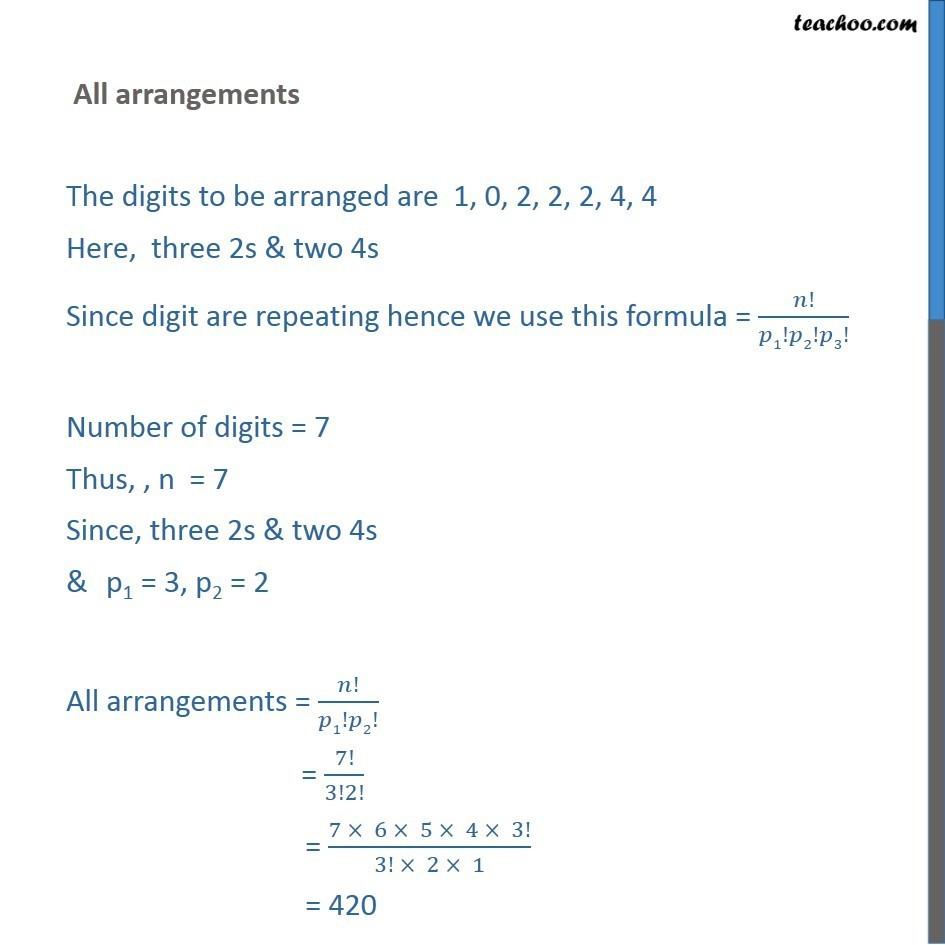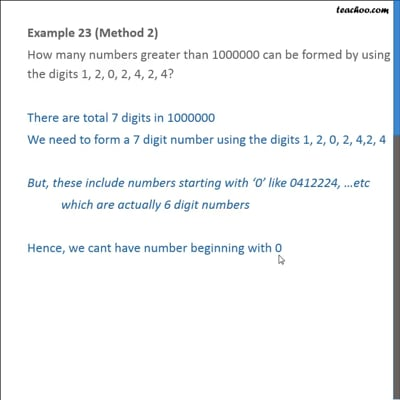This video is only available for Teachoo black users

### Transcript

Example 23 (Method 1) How many numbers greater than 1000000 can be formed by using the digits 1, 2, 0, 2, 4, 2, 4? There are total 7 digits in 1000000 We need to form a 7 digit number using the digits 1, 2, 0, 2, 4, 2, 4 But, these include numbers starting with ‘0’ like 0412224, …etc which are actually 6 digit numbers Hence, we can’t have number beginning with 0 Thus, Required numbers = All arrangements – Numbers starting with 0 All arrangements The digits to be arranged are 1, 0, 2, 2, 2, 4, 4 Here, three 2s & two 4s Since digit are repeating, we use this formula = 𝑛!/(𝑝1! 𝑝2! 𝑝3!) Number of digits = 7 Thus, n = 7 Since, three 2s & two 4s & p1 = 3, p2 = 2 All arrangements = 𝑛!/𝑝1!𝑝2! = 7!/3!2! = (7 × 6 × 5 × 4 × 3!)/(3! × 2 × 1) = 420 If number begins with 0 The remaining digits to be arranged are 1, 2, 2, 2, 4, 4 Here, we have three 2s & two 4s Since digit are repeating, we use this formula = 𝑛!/𝑝1!𝑝2!𝑝3! Number of remaining digit = 6 Thus, n = 6 Since, three 2s & two 4s & p1 = 3, p2 = 2 Thus, Number of numbers beginning with 0 = 𝑛!/𝑝1!𝑝2! = 6!/(3! 2!) = (6 × 5 × 4 × 3!)/(3! × 2 × 1) = 60 Now, Required numbers = All arrangements – Numbers starting with 0 = 420 – 60 = 360 Example 23 (Method 2) How many numbers greater than 1000000 can be formed by using the digits 1, 2, 0, 2, 4, 2, 4? There are total 7 digits in 1000000 We need to form a 7 digit number using the digits 1, 2, 0, 2, 4,2, 4 But, these include numbers starting with ‘0’ like 0412224, …etc. which are actually 6 digit numbers Hence, we cant have number beginning with 0 So the number can begin either with 1, 2 or 4 Thus, Required numbers = Numbers starting with 1 + Numbers starting with 2 + Numbers starting with 4 Thus, Required numbers = Numbers starting with 1 + Numbers starting with 2 + Numbers starting with 4 If number begins with 1 The remaining digits to be arranged are 0, 2, 2, 2, 4, 4 Here, we have three 2s & two 4s Since digit are repeating, we use this formula = 𝑛!/𝑝1!𝑝2!𝑝3! Number of remaining digit = 6 Thus, n = 6 Since, three 2s & two 4s & p1 = 3, p2 = 2 Thus, Number of numbers beginning with 0 = 𝑛!/𝑝1!𝑝2! = 6!/(3! 2!) = (6 × 5 × 4 × 3!)/(3! × 2 × 1) = 60 If number begins with 2 The remaining digits to be arranged are 0, 1, 2, 2, 4, 4 Here, we have two 2s & two 4s Since digit are repeating, we use this formula = 𝑛!/𝑝1!𝑝2!𝑝3! Number of remaining digit = 6 Thus, n = 6 Since, two 2s & two 4s & p1 = 2, p2 = 2 Thus, Number of numbers beginning with 0 = 𝑛!/𝑝1!𝑝2! = 6!/(2! 2!) = (6 × 5 × 4 × 3 × 2!)/(2! × 2 × 1) = 180 If number begins with 4 The remaining digits to be arranged are 0, 1, 2, 2, 2, 4 Here, we have three 2s Since digit are repeating, we use this formula = 𝑛!/𝑝1!𝑝2!𝑝3! Number of remaining digit = 6 Thus, n = 6 Since, three 2s & p1 = 3, Thus, Number of numbers beginning with 0 = 𝑛!/𝑝1!𝑝2! = 6!/3! = (6 × 5 × 4 × 3!)/3! = 120 Thus, Required numbers = Numbers starting with 1 + Numbers starting with 2 + Numbers starting with 4 = 60 + 180 +120 = 360